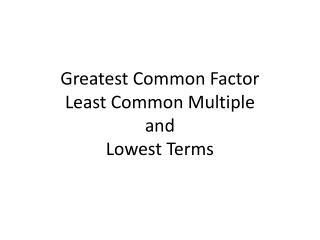DownloadDownload PresentationGreatest Common Factor Least Common Multiple and Lowest Terms

# Greatest Common Factor Least Common Multiple and Lowest Terms

Download Presentation## Greatest Common Factor Least Common Multiple and Lowest Terms

- - - - - - - - - - - - - - - - - - - - - - - - - - - E N D - - - - - - - - - - - - - - - - - - - - - - - - - - -
##### Presentation Transcript

1. Greatest Common FactorLeast Common MultipleandLowest Terms

2. Example 1 find the GCF, LCM and lowest terms of48 and 80 48 and 80 Step 1: set up your upside down division 2 Step 2: find a number that will divide into both numbers 24 and 40 2 Step 3: divide Step 4: repeat until you can’t find any common numbers to divide by 12 and 20 2 6 and 10 2 3 and 5 GCF: multiply the numbers on the outside on the left together LCM: multiply all of the numbers on the outside together Lowest terms: look at the bottom numbers only to make your fraction 3 2 • 2 • 2 •2 = 16 2 • 2 • 2 •2 • 3 • 5 = 240 5

3. Example 2 find the GCF, LCM and lowest terms of208 and 112 208 and 112 Step 1: set up your upside down division 2 Step 2: find a number that will divide into both numbers 104 and 56 2 Step 3: divide Step 4: repeat until you can’t find any common numbers to divide by 52 and 28 2 26 and 14 2 13 and 7 GCF: multiply the numbers on the outside on the left together LCM: multiply all of the numbers on the outside together Lowest terms: look at the bottom numbers only to make your fraction 13 6 = 1 2 • 2 • 2 •2 = 16 2 • 2 • 2 •2 • 7 • 13 = 1456 7 7

4. Example 1 find the GCF, LCM and lowest terms of15 and 32 15 and 32 Step 1: set up your upside down division 1 Step 2: find a number that will divide into both numbers 15 and 32 Step 3: divide Step 4: repeat until you can’t find any common numbers to divide by GCF: multiply the numbers on the outside on the left together LCM: multiply all of the numbers on the outside together Lowest terms: look at the bottom numbers only to make your fraction 15 1 • 15 • 32 = 1 480 32

5. Date _________ Greatest Common FactorLeast Common MultipleandLowest Terms

6. Example 1 find the GCF, LCM and lowest terms of48 and 80 48 and 80 Step 1: set up your Step 2: find a number that will ______ into both numbers Step 3: Step 4: GCF: multiply the numbers on the outside on the left together LCM: multiply all of the numbers on the outside together Lowest terms: look at the bottom numbers only to make your fraction

7. Example 2 find the GCF, LCM and lowest terms of208 and 112 208 and 112 104 and 56 52 and 28 26 and 14

8. Example 3 find the GCF, LCM and lowest terms of15 and 32